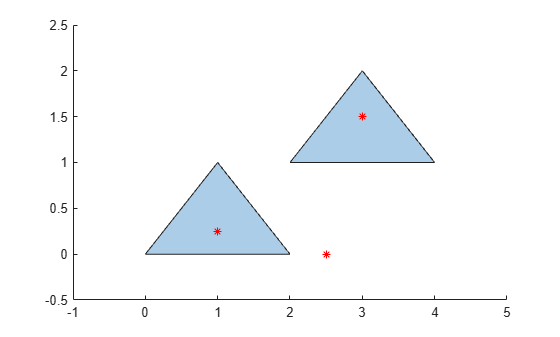# isinterior

Query points inside `polyshape`

## Syntax

``TFin = isinterior(polyin,x,y)``
``TFin = isinterior(polyin,P)``
``[TFin,TFon] = isinterior(___)``

## Description

example

````TFin = isinterior(polyin,x,y)` returns a logical vector whose elements are 1 (`true`) when the corresponding x-coordinates and y-coordinates in the vectors `x` and `y` are in a `polyshape`. A point is in a `polyshape` if it is either in a solid region or on one of the boundaries.```
````TFin = isinterior(polyin,P)` represents the query points in a 2-column matrix `P`. The first column of `P` contains the x-coordinates of the query points, and the second column contains the corresponding y-coordinates.```
````[TFin,TFon] = isinterior(___)` returns an additional logical vector for either of the previous syntaxes. The elements of `TFon` are 1 when the corresponding query points are on a boundary of `polyin`.```

## Examples

collapse all

Create a polygon containing two solid regions.

```x1 = [0 1 2]; y1 = [0 1 0]; x2 = [2 3 4]; y2 = [1 2 1]; polyin = polyshape({x1,x2},{y1,y2}); plot(polyin) hold on```Define three points, and determine if they are in the polygon.

```x = [1 2.5 3]; y = [0.25 0 1.5]; TFin = isinterior(polyin,x,y)```
```TFin = 3x1 logical array 1 0 1 ```
`plot(x,y,'r*')`## Input Arguments

collapse all

Input `polyshape`, specified as a scalar.

Data Types: `polyshape`

x-coordinates of query points, specified as a scalar or vector.

Data Types: `double` | `single`

y-coordinates of query points, specified as a scalar or vector.

Data Types: `double` | `single`

2-D vertices, specified as a 2-column matrix. The first column of `P` contains the x-coordinates of the query points, and the second column contains the y-coordinates.

Data Types: `double` | `single`

## Output Arguments

collapse all

Interior point indicator, returned as a scalar or vector. An element of `TFin` is 1 when the corresponding query point is either in a solid region of the input `polyshape` or on a boundary.

Data Types: `logical`

Boundary point indicator, returned as a scalar or vector. An element of `TFon` is 1 when the corresponding query point is strictly on a boundary of the `polyshape`.

Data Types: `logical`

## Version History

Introduced in R2017b